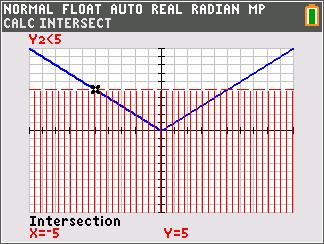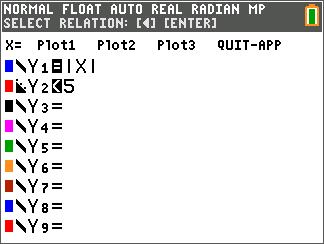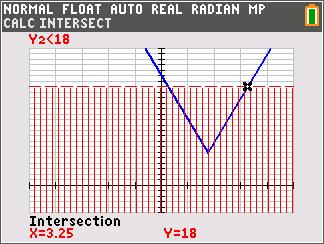# Activities

••• ##### Subject Area

• Math: Algebra I: Absolute Value Functions
• Math: Algebra I: Equations and Inequalities

• ##### Author9-12

45 Minutes

• ##### Device
• TI-84 Plus
• TI-84 Plus Silver Edition
•TI-84 Plus C Silver Edition
•TI-84 Plus CE
• ##### Software

TI Connect™ CE

## Can I Graph You, Too?#### Activity Overview

Students explore absolute value inequalities graphically, numerically, and algebraically.

#### Key Steps

•This activity is intended to be an introductory exploration into solving absolute value inequalities through graphing. The method of graphing is compared to the method used for finding the solution to an absolute value equation (graphing both sides and finding the intersection points).

•They are given the definition and graphical examples of disjunction and conjunction using the Inequalz App. A teacher demonstration may be required for students to fully understand the difference between the two terms.

•Students will first write the inequality given as a conjunction or disjunction and then solve for the absolute value They will then graph the left side and the right side and find the intersection points. The inequality symbol will tell students if the intersection points are included or not included in the solution.